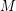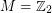# Cores of Cayley graphs

 Importance: Medium ✭✭
 Author(s): Samal, Robert
 Subject: Graph Theory » Coloring » » Homomorphisms
 Keywords: Cayley graph core
 Posted by: Robert Samal on: March 6th, 2007
Conjecture   Letbe an abelian group. Is the core of a Cayley graph (on some power of) a Cayley graph (on some power of)?

Even the caseis open. In this case, Cayley graphs on some power ofare called cube-like graphs, they have been introduced by Lov\'asz as an example of graphs, for which every eigenvalue is an integer.

So, in this case we ask, whether a core of each cube-like graph is a cube-like graph.

### Who first conjectured this?

Who first conjectured that the core of a cubelike graph is cubelike?

### Question needs refining...

As stated, the conjecture is false in an uninteresting way ... it is possible for a Cayley graph of Z_15 have a 5-cycle as a core.... So if we take M = Z_15 then the result is false.

Perhaps the question should either (a) be restricted to elementary abelian groups or (b) have the conclusion being that the core of a Cayley graph on M must be a Cayley graph on N where N is a (group) homomorphic image of M.

Gordon Royle http://people.csse.uwa.edu.au/gordon

### Re: Question needs refining...

That is true. I was mainly thinking about(for a prime). However, your suggestion (b) looks sensible as well.